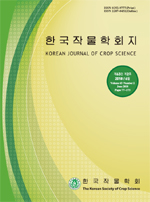상세검색
최근 검색어 전체 삭제
다국어입력
즐겨찾기0KCI등재 학술저널

# 밀 유전자원의 근적외선분광분석 예측모델에 의한 단백질 함량 변이분석Statistical Analysis of Protein Content in Wheat Germplasm Based on Near-infrared Reflectance Spectroscopy

• 등재여부 : KCI등재
• 2019.12
• 353 - 365 (13 pages)

A near-infrared reflectance spectroscopy (NIRS) prediction model was set to establish a rapid analysis system of wheat germplasm and provide statistical information on the characteristics of protein contents. The variability index value (VIV) of calibration resources was 0.80, the average protein content was 13.2%, and the content range was from 7.0% to 13.2%. After measuring the near-infrared spectra of calibration resources, the NIRS prediction model was developed through a regression analysis between protein content and spectra data, and then optimized by excluding outliers. The standard error of calibration, R2, and the slope of the optimized model were 0.132, 0.997, and 1.000 respectively, and those of external validation results were 0.994, 0.191, and 1.013, respectively. Based on these results, a developed NIRS model could be applied to the rapid analysis of protein in wheat. The distribution of NIRS protein content of 6,794 resources were analyzed using a normal distribution analysis. The VIV was 0.79, the average protein was 12.1%, and the content range of resources accounting for 42.1% and 68% of the total accessions were 10-13% and 9.5-14.6%, respectively. The composition of total resources was classified into breeding line (3,128), landrace (2,705), and variety (961). The VIV in breeding line was 0.80, the protein average was 11.8%, and the contents of 68% of total resources ranged from 9.2% to 14.5%. The VIV in landrace was 0.76, the protein average was 12.1%, and the content range of resources of 68% of total accessions was 9.8-14.4%. The VIV in variety was 0.80, the protein average was 12.8%, and the accessions representing 68% of total resources ranged from 10.2% to 15.4%. These results should be helpful to the related experts of wheat breeding.

재료 및 방법

결과 및 고찰

적요

사사

인용문헌(REFERENCES)